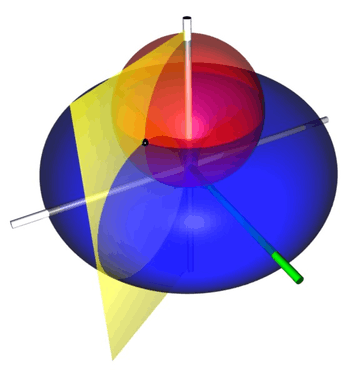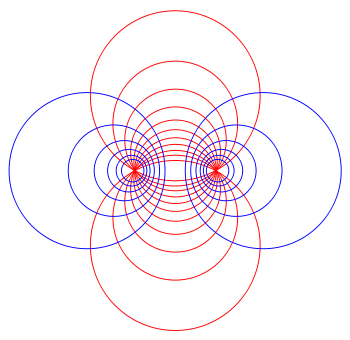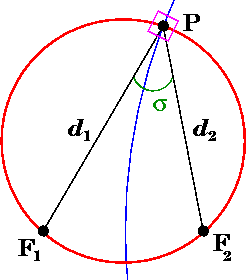# Toroidal coordinatesIllustration of toroidal coordinates, which are obtained by rotating a two-dimensional bipolar coordinate system about the axis separating its two foci. The foci are located at a distance 1 from the vertical z-axis. The red sphere is the σ = 30° isosurface, the blue torus is the τ = 0.5 isosurface, and the yellow half-plane is the φ = 60° isosurface. The green half-plane marks the x-z plane, from which φ is measured. The black point is located at the intersection of the red, blue and yellow isosurfaces, at Cartesian coordinates roughly (0.996, 1.725, 1.911).

Toroidal coordinates are a three-dimensional orthogonal coordinate system that results from rotating the two-dimensional bipolar coordinate system about the axis that separates its two foci. Thus, the two fociandin bipolar coordinates become a ring of radiusin theplane of the toroidal coordinate system; the-axis is the axis of rotation. The focal ring is also known as the reference circle.

## Definition

The most common definition of toroidal coordinatesiswhere thecoordinate of a pointequals the angleand thecoordinate equals the natural logarithm of the ratio of the distancesandto opposite sides of the focal ringThe coordinate ranges areandand### Coordinate surfacesRotating this two-dimensional bipolar coordinate system about the vertical axis produces the three-dimensional toroidal coordinate system above. A circle on the vertical axis becomes the red sphere, whereas a circle on the horizontal axis becomes the blue torus.

Surfaces of constantcorrespond to spheres of different radiithat all pass through the focal ring but are not concentric. The surfaces of constantare non-intersecting tori of different radiithat surround the focal ring. The centers of the constant-spheres lie along the-axis, whereas the constant-tori are centered in theplane.

### Inverse transformation

The (σ, τ, φ) coordinates may be calculated from the Cartesian coordinates (x, y, z) as follows. The azimuthal angle φ is given by the formulaThe cylindrical radius ρ of the point P is given byand its distances to the foci in the plane defined by φ is given byGeometric interpretation of the coordinates σ and τ of a point P. Observed in the plane of constant azimuthal angle φ, toroidal coordinates are equivalent to bipolar coordinates. The angle σ is formed by the two foci in this plane and P, whereas τ is the logarithm of the ratio of distances to the foci. The corresponding circles of constant σ and τ are shown in red and blue, respectively, and meet at right angles (magenta box); they are orthogonal.

The coordinate τ equals the natural logarithm of the focal distanceswhereas the coordinate σ equals the angle between the rays to the foci, which may be determined from the law of cosineswhere the sign of σ is determined by whether the coordinate surface sphere is above or below the x-y plane.

### Scale factors

The scale factors for the toroidal coordinatesandare equalwhereas the azimuthal scale factor equalsThus, the infinitesimal volume element equalsand the Laplacian is given byOther differential operators such asandcan be expressed in the coordinatesby substituting the scale factors into the general formulae found in orthogonal coordinates.

## Toroidal harmonics

### Standard separation

The 3-variable Laplace equationadmits solution via separation of variables in toroidal coordinates. Making the substitutionA separable equation is then obtained. A particular solution obtained by separation of variables is:where each function is a linear combination of:Where P and Q are associated Legendre functions of the first and second kind. These Legendre functions are often referred to as toroidal harmonics.

Toroidal harmonics have many interesting properties. If you make a variable substitutionthen, for instance, with vanishing order (the convention is to not write the order when it vanishes) andandwhereandare the complete elliptic integrals of the first and second kind respectively. The rest of the toroidal harmonics can be obtained, for instance, in terms of the complete elliptic integrals, by using recurrence relations for associated Legendre functions.

The classic applications of toroidal coordinates are in solving partial differential equations, e.g., Laplace's equation for which toroidal coordinates allow a separation of variables or the Helmholtz equation, for which toroidal coordinates do not allow a separation of variables. Typical examples would be the electric potential and electric field of a conducting torus, or in the degenerate case, an electric current-ring (Hulme 1982).

### An alternative separation

Alternatively, a different substitution may be made (Andrews 2006)whereAgain, a separable equation is obtained. A particular solution obtained by separation of variables is then:where each function is a linear combination of:Note that although the toroidal harmonics are used again for the T  function, the argument israther thanand theandindices are exchanged. This method is useful for situations in which the boundary conditions are independent of the spherical angle, such as the charged ring, an infinite half plane, or two parallel planes. For identities relating the toroidal harmonics with argument hyperbolic cosine with those of argument hyperbolic cotangent, see the Whipple formulae.

## Bibliography

• Morse P M, Feshbach H (1953). Methods of Theoretical Physics, Part I. New York: McGraw–Hill. p. 666.
• Korn G A, Korn T M (1961). Mathematical Handbook for Scientists and Engineers. New York: McGraw-Hill. p. 182. LCCN 59014456.
• Margenau H, Murphy G M (1956). The Mathematics of Physics and Chemistry. New York: D. van Nostrand. pp. 190192. LCCN 55010911.
• Moon P H, Spencer D E (1988). "Toroidal Coordinates (η, θ, ψ)". Field Theory Handbook, Including Coordinate Systems, Differential Equations, and Their Solutions (2nd ed., 3rd revised printing ed.). New York: Springer Verlag. pp. 112115 (Section IV, E4Ry). ISBN 0-387-02732-7.